# ML Aggarwal Class 8 Solutions for ICSE Maths Chapter 5 Playing with Numbers Ex 5.1

## ML Aggarwal Class 8 Solutions for ICSE Maths Chapter 5 Playing with Numbers Ex 5.1

Question 1.
Write the following numbers in the generalized form:
(i) 89
(ii) 207
(iii) 369
Solution: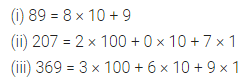Question 2.
Write the quotient, when the sum of a 2-digit number 34 and number obtained by reversing the digits is divided by
(i) 11
(ii) sum of digits
Solution: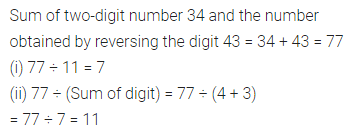Question 3.
Write the quotient when the difference of a 2-digit number 73 and number obtained by reversing the digits is divided by
(i) 9
(ii) the difference of digits.
Solution: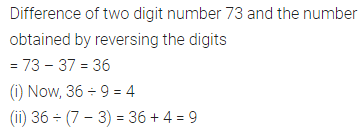Question 4.
Without actual calculation, write the quotient when the sum of a 3-digit number abc and the number obtained by changing the order of digits cyclically i.e. bca and cab is divided by
(i) 111
(ii) (a + b + c)
(iii) 37
(iv) 3
Solution:Question 5.
Write the quotient when the difference of a 3-digit number 843 and number obtained by reversing the digits is divided by
(i) 99
(ii) 5
Solution: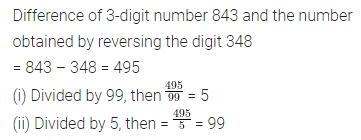Question 6.
The sum of digits of a 2-digit number is 11. If the number obtained by reversing the digits is 9 less than the original number, find the number.
Solution:Question 7.
If the difference of a two-digit number and the number obtained by reversing the digits is 36, find the difference between the digits of the 2-digit number.
Solution: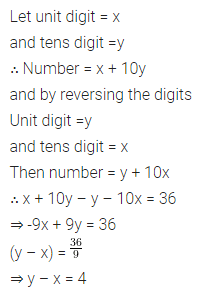Question 8.
If the sum of two-digit number and number obtained by reversing the digits is 55, find the sum of the digits of the 2-digit number.
Solution: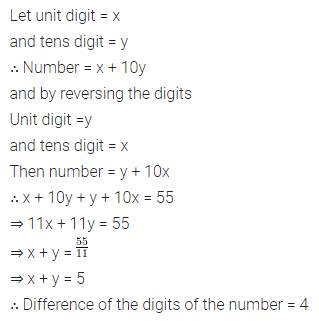Question 9.
In a 3-digit number, unit’s digit, ten’s digit and hundred’s digit are in the ratio 1 : 2 : 3. If the difference of the original number and the number obtained by reversing the digits is 594, find the number.
Solution:Question 10.
In a 3-digit number, the unit’s digit is one more than the hundred’s digit and ten’s digit is one less than the hundred’s digit. If the sum of the original 3-digit number and numbers obtained by changing the order of digits cyclically is 2664, find the number.
Solution: